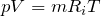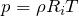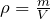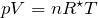# Ideal Gas Law

Ideal Gas Law

An ideal, or perfect, gas exists where the kinetic energy of its molecules are dependant only on temperature. It therefore would obey gas laws (e.g. Boyle’s Law & Charles’s Law) exactly at all pressures and temperatures.

The ideal gas law is a combination of Charles’s Law and Boyle’s Law and is the equation of state that exists for such an ideal gas. Many gases (e.g. air, nitrogen, oxygen, hydrogen etc.) can be treated as an ideal gas.

Ideal Gas Law in Relation to Individual Gas Constant Ri

 Formula:p = absolute pressure (N/m², lb/ft²) V = volume (m³, ft³) m = mass (kg, slugs) Ri = individual gas constant (J/Kg.K, ft.lb/slugs.°R) T = temperature (K; °R)

This can be alternatively written in relation to density, ρ, as:

 Formula:Ideal Gas Law in Relation to Universal Gas Constant R*

 Formula:p = absolute pressure (N/m², lb/ft²) V = volume (m³, ft³) n = number of moles of gas R* = universal gas constant (J/mol.K, ft.lb/lb-mol.°R) T = temperature (K; °R)